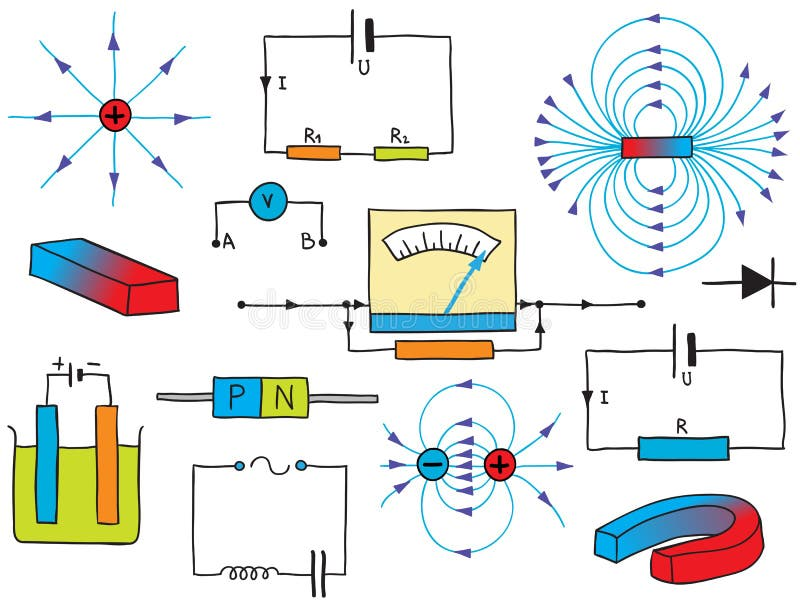•

Unit 5: Electricity and Magnetism

Long Term Learning Targets:

I can calculate and determine the electric forces between two charged objects by applying Coulomb’s law. I can verify the Ohm's law by measuring the current and voltage in a circuit and I can analyze the circuit with resistors in series and in parallel by applying the Ohm’s law. I can determine the electric energy and power in an electric circuit by the derived formulas during analyzing the electric circuit. I can understand the magnetic fields creating from moving charges through a conductor by the applying the Ampere’s law. And I can connect the relative motion between a conductor and a magnetic field to an induced electric potential difference by the electromagnetic induction as stated in the Faraday’s law.